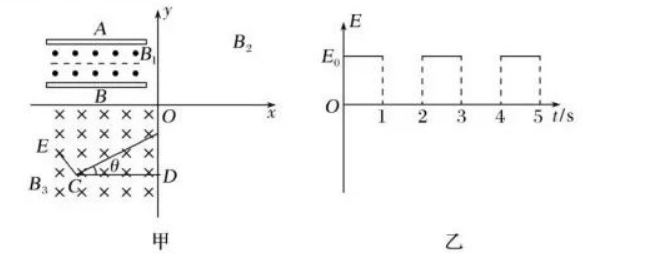(1)求称定后两金属板之间的电势差 $U_{A B}$ :
(2)求带电粒子在第一象限磁场中做圆周运动的半径 $r_2$ :
(3)求第一象限内磁场的最小面积 $S_{\min }$ 与斜面倾角 $\theta$ 的函数关系式;
(4)若带电粒子在第 $19 \mathrm{~s}$ 内恰好没有㼘开斜面, $19 \mathrm{~s}$ 后电场变为垂直斜面向上的匀强电 场, 电场大小变为 $\frac{3}{4} E_0 \cos \theta$, 并在斜面末端安装一垂直斜面的菼光屏 $C E$ 。已知带电粒子在 电场变化后的 $\frac{1}{4} \mathrm{~s}$ 内打在䓎光屏上, 且与 $C$ 点的距离为 $\frac{q E_0 \cos \theta}{32 \pi^2 m}$, 求 $19 \mathrm{~s}$ 末带电粒子与斜面 底端 $C$ 点的距离 $L$ (计算结果用角度关系表示)。